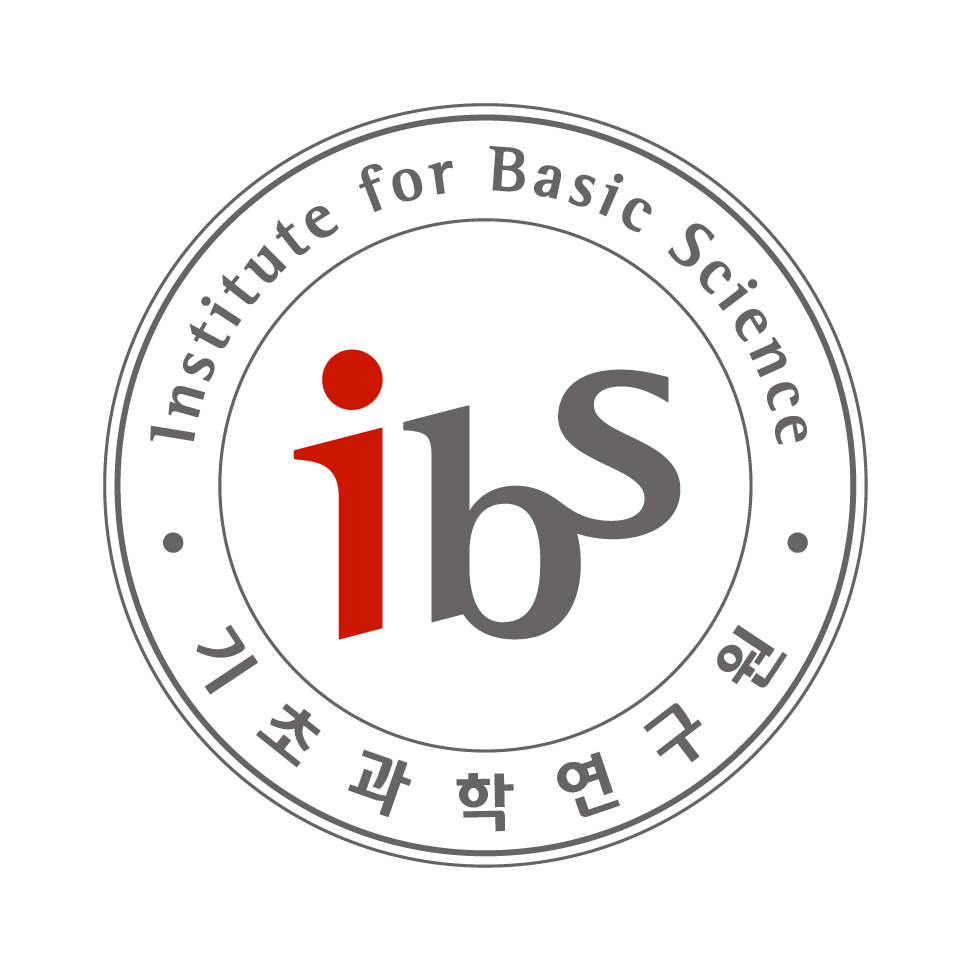# The 3rd Mini Workshop on Knot theory

## Low dimensional topology

June 16–17, 2017

Korea University, Seoul, Korea

sponsered by

## Mini-Course Lecturer

• Sangbum Cho (Hanyang University)
• Kyungbae Park (KIAS)

## Speakers

• Seonhwa Kim (IBS-CGP)
• Bo-hyun Kwon (Korea University)
• Hyunshik Shin (KAIST)

## Organizers

• Byung Hee An (IBS-CGP)
• Hwa Jeong Lee (DGIST)
• Jung Hoon Lee (Chonbuk National University)

## Local Organizer

• Seungsang Oh (Korea University)

## Talk Schedule

June 16 (Fri) June 17 (Sat)
09:30 – 10:15 Arrival Hyunshik Shin
10:15 – 10:45 Coffee Break
10:45 – 11:30 Bo-hyun Kwon
11:45 – 12:30 Seonhwa Kim
12:30 – 14:00 Lunch & Departure
14:00 – 14:45 Sangbum Cho I
15:00 – 15:45 Sangbum Cho II
15:45 – 16:15 Coffee Break
16:15 – 17:00 Kyungbae Park I
17:15 – 18:00 Kyungbae Park II
18:00 – Dinner

## Title & Abstract

Speaker
Sangbum Cho (Hanyang University)
Title
Disk complexes, mapping class groups, and 2-bridge knots
Abstract
We describe the combinatorial structure of the disk complex of a genus-2 handlebody. In particular, when the handlebody is one of the handlebodies of a reducible genus-2 Heegaard splitting, the disk complex admits an interesting subcomplex, called the primitive disk complex. As applications of a study of the primitive disk complexes, first we provide a finite presentation of the mapping class group of each of the reducible genus-2 Heegaard splittings, and next we give an alternative proof of a result of Kobayashi and Saeki that every (1, 1)-position of a non-trivial 2-bridge knot is a stabilization of its 2-bridge position. This is a joint work with Yuya Koda.
Speaker
Seonhwa Kim (IBS-CGP)
Title
Partially abelian PSL(2,C) representations of knot groups
Abstract
Octahedral developings of knot complement are inspired by Volume conjecture. These are parametrized by several ways using complex variables related to cross-ratios, in particular segment variables and region variables. We will see a condition if there is a missing representation in a solution set of gluing equation and introduce the notion of partially abelian representation, which is also related to a PSL(2,C) representation of virtually knot group.

Speaker
Bo-hyun Kwon (Korea University)
Title
Introduction to trisection and bridge trisection
Abstract
In this talk, we introduce the Trisection of closed, smooth 4-manifolds which was developed by Gay and Kirby and the Bridge Trisection of knotted surfaces in $S^4$ which is introduced by Meier and Zupan. Also, I would give some interesting open problems about these topics.
Speaker
Kyungbae Park (KIAS)
Title
Heegaard Floer correction terms, definite 4-manifolds, and Dehn surgery
Abstract
Heegaard Floer correction term is a rational-valued invariant for closed, oriented 3-manifolds equipped with torsion $spin^c$ structures, introduced by Ozsváth and Szabó using the absolute grading of Heegaard Floer homology. In particular, it is known that the invariant gives constraints on definite smooth 4-manifolds bounded by a give 3-manifold. In this talk, we discuss the construction and the formal properties of the correction term, and introduce two applications of it. First, we present infinitely many examples of closed, oriented, irreducible 3-manifolds $M$ such that $b_1(M)=1$ and $\pi_1(M)$ has weight 1, but $M$ is not the result of Dehn surgery along a knot in the 3-sphere. This is a joint work with Matt Hedden and Min Hoon Kim. Secondly, we show if a rational homology 3-sphere $Y$ bounds a positive definite smooth 4-manifold, then there are only finitely many intersection forms of negative definite smooth 4-manifolds bounded by $Y$. This is a joint work with Dong Heon Choe.

Speaker
Hyunshik Shin (KAIST)
Title
Small asymptotic translation lengths of pseudo-Anosov maps on the curve complex
Abstract
Let $M$ be a hyperbolic fibered 3-manifold with $b_1(M) \geq 2$ and let $S$ be a fiber with pseudo-Anosov monodromy $\psi$. We show that there exists a sequence $(R_n, \psi_n)$ of fibers contained in the fibered cone of $(S,\psi)$ such that the asymptotic translation length of $\psi_n$ on the curve complex $\mathcal{C}(R_n)$ behaves asymptotically like $1/|\chi(R_n)|^2$. As an application, we can reprove the previous result by Gadre--Tsai that the minimal asymptotic translation lengths of a closed surface of genus $g$ are bounded below and above by $C/g^2$ and $D/g^2$ for some positive constants $C$ and $D$, respectively. We also show that this also holds for the cases of hyperelliptic mapping class group and hyperelliptic handlebody group.

## Registration

Online registration is available here until June 7, 2017.

## Contact

Byunghee An (anbyhee_at_ibs.re.kr)

## Previous Editions